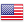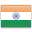Grade "A+" Accredited by NAAC with a CGPA of 3.46
Grade "A+" Accredited by NAAC with a CGPA of 3.46

# Financial Analytics

Course ID
FS 401
Level
Program
BBA (FIA)
Semester
Fourth
Credits
4.0
Paper Type
Skill Enhancement
Method
Lecture & Practical

## Unique Paper Code: Update Awaited

• To give introduction of machine learning techniques to students
• To provide knowledge of Python programming

## Learning Outcomes:

At the end of the course, students should be able to:

• Students will learn basics of Machine learning
• Students will understand Python programming language.
• Students will be able to apply these techniques on financial data

## Course Contents

Unit I
Unit II
Unit III
Unit IV

Unit I (4 weeks)

Data Manipulation using Python: Introduction to Python, IDE’s (Jupyter, Spyder), custom environment settings, Data Structure: basic data types (numeric, string, float, date timestamp), aggregate functions, conditions (if-elif-else), looping (for, while), inbuilt functions for data conversion, writing user defined functions. Concepts of packages/libraries – important packages like NumPy, scikit-learn, scipy, sympy, math, Pandas, Matplotlib, etc. importing packages using pip, reading and writing data from/to different formats: Data frame, arrays, list of list, series, sets, dictionaries, plotting, functions, list comprehensions (index comprehension). Application of machine learning algorithm for solving problem in financial markets.

References:

Dive into PYTHON 3 by Mark Pilgrim : Chapters 1, 2, 4

Python Machine Learning by Sebastian Raschka: Chapter 3

Unit II (2 weeks)

Machine learning: Introduction, Definitions, Supervised, unsupervised, python libraries for machine learning, Sci-kit learn, Applications of Machine learning in Financial Technology (FinTech).

References:

Machine Learning by Tom M. Mitchell: Chapter 1, 2, 4

Unit III (2 weeks)

Regression: Linear regression univariate and multivariate, nonlinear regression, over-fitting and regularization, logistic regression, Case studies based on regression techniques (using financial market data)

References:

An Introduction to Statistical Learning by Gareth James: Chapter 3 and Chapter 4

Unit IV (3 weeks)

Classification: K Neighbors, K – means, decision Trees and SVM.

Clustering: Partial based clustering, hierarchical clustering, intensity based clustering, Neural Network: Single layer perceptron, multi-layer perceptron, back propagation algorithm applying neural network on financial market data

References:

Machine Learning by Tom M. Mitchell: Chapter 3, 4, 6, 8

### Practicals

#### Lab List 1

The entire syllabus is based on practical exercise i.e. learning through doing in computer lab on specified SW like Python and Matlab. The exam will also have 50% weightage for practical in the lab with evaluation through external expert.

#### Text Books

Dive into PYTHON 3 by Mark Pilgrim
Machine Learning by Tom M. Mitchell
An Introduction to Statistical Learning by Gareth James

Step-by-Step Machine Learning with Python By Yuxi (Hayden) Liu
Regression Analysis with Python By Luca Massaron, Alberto Boschetti
Principles of Soft Computing, by S. N. Deepa and S. N. Sivanandam
Python Machine Learning by Sebastian Raschka

#### Teaching Learning Process

Class room lecture, Practical Lab Session, Problem solving, Class presentation on the assigned topic by students individually or in group, Workshop

#### Assessment Methods

1. Practical exam of 50% marks inclusive of internal evaluation (25%). Internal evaluation will have:
a. Attendance 5% marks
b. Two internal evaluations by the teacher with 10% marks each out of which one must be a class test and other may be another test or home assignment or presentation. Faculty may take more than two assignments and (or) tests but total will be only 20% marks.
2. End term University Exam of 50% marks

#### Keywords

Data Structure, Python, Machine learning, Neural Network, Regression

Disclaimer: Details on this page are subject to change as per University of Delhi guidelines. For latest update in this regard please refer to the University of Delhi website here.Englishहिन्दी